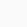# How to use square root(√x) symbol in LaTeX?

Symbol/UnicodeSquare root/U+221A
Type of symbolMathematics
Package (requirement)No
Argumentyes, x
Latex command\sqrt{x}
Example\sqrt{x} → √x

Many times in mathematical equations we need a Square root symbol, it is very easy to use in latex. To print this symbol in a latex document you need to use \sqrt{arg} command and also pass an argument with it.

\documentclass{article}
\begin{document}
$\sqrt{x}$
$x=\frac{-b\pm\sqrt{b^2-4ac}}{2a}$
$\frac{12}{\sqrt{15}-\sqrt{7}}\cdot\frac{\sqrt{15}+\sqrt{7}}{\sqrt{15}+\sqrt{7}}$
\end{document}

Output :Scroll to Top